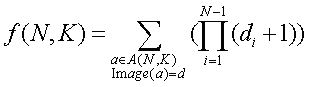Online JudgeProblem SetAuthorsOnline ContestsUser
Web Board
F.A.Qs
Statistical Charts
Problems
Submit Problem
Online Status
Prob.ID:
Register
Authors ranklist
Current Contest
Past Contests
Scheduled Contests
Award Contest
Register
Language:
A Difficult Mathematics Problem
 Time Limit: 2000MS Memory Limit: 65536K Total Submissions: 404 Accepted: 139

Description

Here is a difficult mathematics problem; your job is to solve it.

Given two integer N and K, we have
A(N, K) = {a | a = (a1 a2 a3 … aN), ai is integer and 0 <= ai <= K-1, i = 1,...,N}.

We define a function d = Image(a) from A(N, K) to A(N-1, K) as below:
For any a = (a1 a2 a3 ... aN), which is belonged to A(N, K), we have d = (d1 d2 ... dN-1) = Image(a) with di = min(ai, ai+1), i.e., di is the smaller one between ai and ai+1.

You should calculate the expression belowFor any element a belonged to A(N, K), the expression first obtains d = (d1 d2 ... dN-1) = Image(a); then gain a value by calculating (d1+1)(d2+1)...(dN-1+1). Each a corresponding to a value, and f(N, K) is just the sum of these values.

For example, If N = 2, K = 3, then A(N, K)={(00), (01), (02), (11), (10), (12), (20), (21), (22)}; the values obtained from each element in A(N, K) are 1, 1, 1, 2, 1, 2, 1, 2, 3 and we can know that f(2, 3) = 14 by adding these values.

Input

The input consists of only one line, which contains two integer N and K (N = 2, 3, 4. And if N=2, we has 1 <= K <= 5000; if N=3, we has 1 <= K <= 1000; if N=4, we has 1 <= K <= 500).

Output

The output contains a single integer, which is the value of f(N, K). It's confirmed that the result is less than 2^63.

Sample Input

`2 3`

Sample Output

`14`

Source

POJ Monthly,李学武

[Submit]   [Go Back]   [Status]   [Discuss]Home PageGo BackTo top